# Gravitational Field Formula

Gravitational Field Formula

The acceleration due to gravity near the Earth depends on the distance of an object from Earth's center. The gravitational field formula can be used to find the field strength, meaning the acceleration due to gravity at any position around the Earth. The radius of the Earth is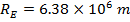, and so values of r in the formula are (typically) greater than this radius. The gravitational field strength is measured in Newtons per kilogram (), or in the same units as acceleration,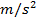.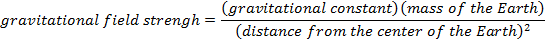g(r) = Earth's gravitational field strength (or)

G = gravitational constant (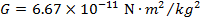)

mE = mass of the Earth (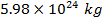)

r = distance from the center of the Earth (m)

Gravitational Field Formula Questions:

1) What is the gravitational field strength at the surface of the Earth,?

Answer: The gravitational field strength at the surface of the Earth can be calculated using the formula:The value of r of interest is the radius of the Earth,: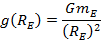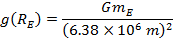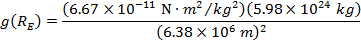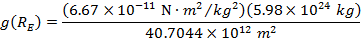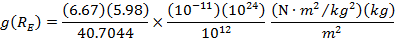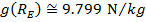The gravitational field strength at the Earth's surface is approximately. This is equivalent to an acceleration due to gravity at the Earth's surface of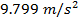.

2) Some satellites orbit at a distance that puts them in what is called geosynchronous orbit. At this distance, the satellites orbit once per day, and so they stay above a fixed point on the Earth. Geosynchronous orbits have a radius of. What is the gravitational field strength (and acceleration due to gravity) at geosynchronous orbit?

Answer: The gravitational field strength at the geosynchronous orbital radius can be calculated using the formula:The value of r of interest is: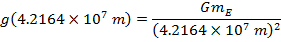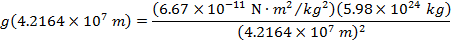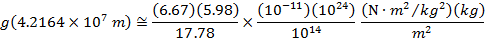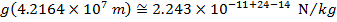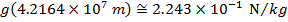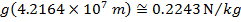The gravitational field strength at the geosynchronous orbital radius is approximately. This is equivalent to an acceleration due to gravity of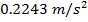.